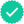Question

Figure a is a partial graph of the position function x(t) for a simple harmonic oscillator with an angular frequency of 1.40 rad/s; figure b is a partial graph of the corresponding velocity function v(t). The vertical axis scales are set by xs = 7.50 cm and vs = 7.50 cm/s. What is the phase constant (from -π to π rad) of the SHM if the position function x(t) is given the form x = xm cos(ωt + φ)?

Expert SolutionKnowledge Booster••